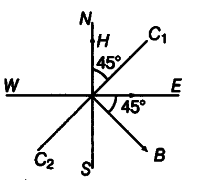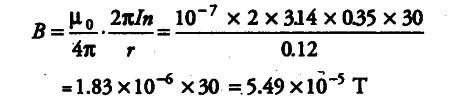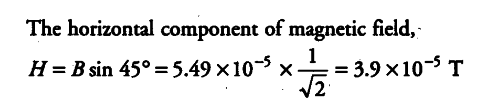# A compass needle free to turn in a horizontal plane

A compass needle free to turn in a horizontal plane is placed at the centre of circular coil of 30 turns and radius 12 cm. The coil is in a vertical plane making an angle of 45° with the magnetic meridian. When the current in the coil is 0.35 A, the needle points West to East.
(i) Determine the horizontal component of the earth’s magnetic field at the location.
(ii) The current in the’ coil is reversed and the coil is rotated about its vertical axis by an angle of 90° in the anti-clockwise sense looking from above. Predict the direction of the needle. Take the magnetic declination at the places to be zero.

Given, number of turns in the coil, w = 30
Current in the coil, / = 0.35A Radius of circular coil,
r = 12 cm = 0.12 m (i) Let N-S be the line of magnetic meridian, the coil is placed at an angle of 45° with the magnetic meridian the planes of coil. The needle point towards from West to East. The magnetic field produced due to the coil is B and the direction of magnetic field B is along the axis of coil, i.e. it makes an angle of 45° with East. The needle points towards from West to East only if the direction of magnetic field B is at an angle 45° from East.
Use the formula of magnetic field produced by a current carrying coil,As the direction of current in the coil is reversed and the coil is turned by 90° anti-clockwise, the direction of needle will reverse, i.e. it points from East to West.